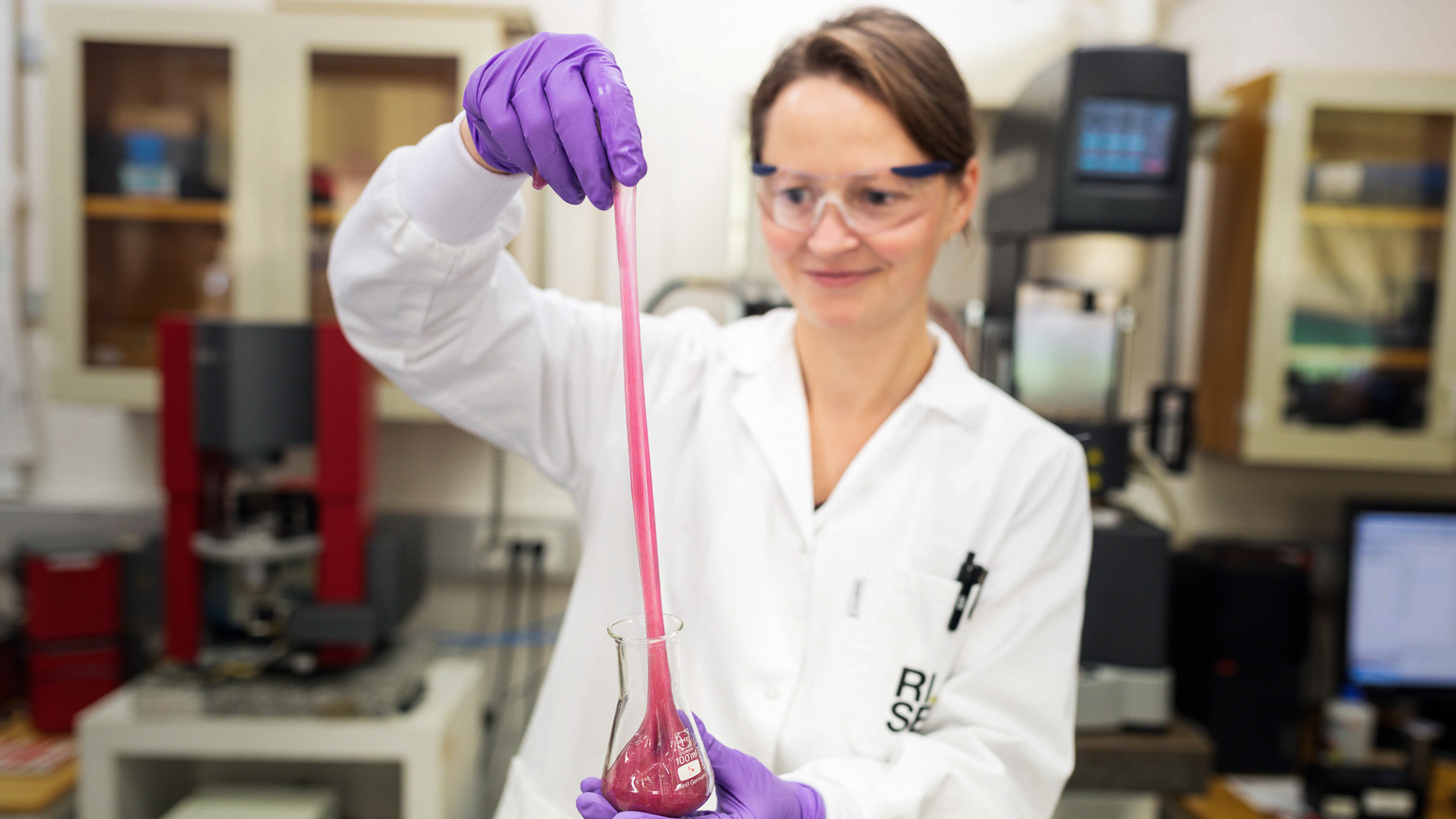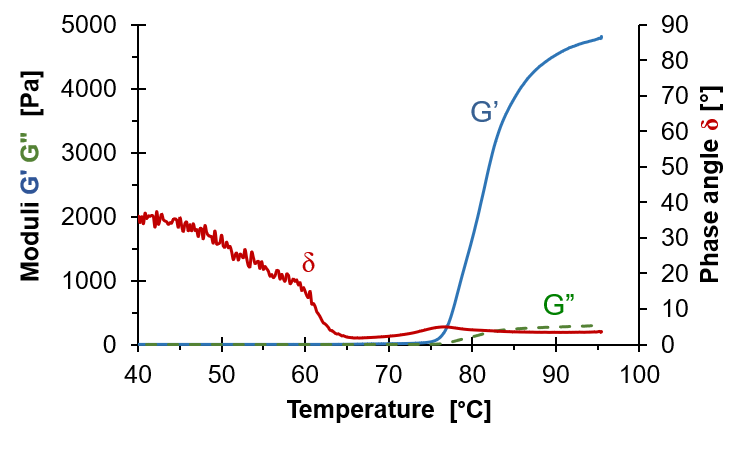# Introduction to RheologyThe ketchup will not come out of the bottle, the concrete does not fill the mould or the paint drips. These are all problems which can be explained and solved by rheology. Rheology is defined as the study of flow of matter and deals with liquids and semi-solid fluids. Rheology covers all types of fluids ranging from food and plastics to mud and glaciers. The common denominator is that the fluids behave intermediate in between a thin fluid and a solid.

#### Viscous flow

One of the properties often dealt with in rheology is viscosity which measures how thick a fluid is, or more precise its resistance to flow. A viscous fluid thus has a higher viscosity than a thinner fluid, such as honey compared with water. In most cases, we deform a fluid in shear, like the flow in a pipe, but a fluid has an even greater resistance to stretching. The extension occurs when we try to squeeze the fluid through a contraction, like when we swallow or force a fluid out of a nozzle.

The resistance to flow depends on how fast we deform (shear or extend) the fluid rather on how much we deform it, as is expressed by the deformation rate. We express the resistance in stress which is force per unit area, and the rate in shear as the dimensionless shear rate. The shear viscosity η is then η=shear stress/shear rate.

The shear rate varies depending on the process. In slow flows like sedimentation the shear rate is low, typically ≤10-4 s-1. But it is high in processes involving flow in thin layers, such as when we apply skin cream or coat paper, typically ≥104. Viscosity also varies greatly – from water (10-3 Pa·s at 20°C) to molten plastic (103 Pa·s) and asphalt (108 Pa·s). The viscosity depends on a range of parameters such shear rate, time and temperature which is further treated in the extended introduction.

#### Viscoelasticity

Some materials are intermediate between solids and fluids and viscosity alone is then not enough to characterize the material. In the photo, rheologist Johanna Andersson demonstrates viscoelasticity with slime which flows (is viscous) and at the same time is resilient (elastic). As an example, viscosity is adequate for describing the state of egg white when raw. But when the egg is boiled and solidifies, viscosity becomes irrelevant for describing the consistency of the boiled egg as it goes to infinity. We then need viscoelasticity.

In linear viscoelasticity a small harmonic deformation or stress is applied to a fluid and the resulting response is monitored. This common characterisation method is referred to as small amplitude oscillatory shear (SAOS) and is excellent for monitoring transitions as the small deformations do not break any structure of the fluid. The monitored data is converted to a complex shear modulus G* consisting of two parts, the storage modulus G’ and the loss modulus G”. The relation between them is expressed through the phase angle δ as tan δ=G”/G’. One can use any two of these parameters, for example G’ expressing the fluid stiffness and δ the character of the fluid (δ=0 means solid and δ=90° means fluid).The diagram shows SAOS results for boiling egg white. At low temperature both G’ and G” are small as the egg white then flows easily. It is however a bit slimy demonstrated by the phase angle δ which is far from 90°. When temperature increases δ drops as the egg white solidifies and G’ and G” increase. The storage modulus G’ increases more than G” displaying the elastic nature of the boiled egg white.

If you want to dig deeper into rheology an on-line textbook Introduction to rheology for educated beginners" is available. Also plenty in depth textbooks are available and three of them are